# 18: Solutions

$$\newcommand{\vecs}{\overset { \rightharpoonup} {\mathbf{#1}} }$$ $$\newcommand{\vecd}{\overset{-\!-\!\rightharpoonup}{\vphantom{a}\smash {#1}}}$$$$\newcommand{\id}{\mathrm{id}}$$ $$\newcommand{\Span}{\mathrm{span}}$$ $$\newcommand{\kernel}{\mathrm{null}\,}$$ $$\newcommand{\range}{\mathrm{range}\,}$$ $$\newcommand{\RealPart}{\mathrm{Re}}$$ $$\newcommand{\ImaginaryPart}{\mathrm{Im}}$$ $$\newcommand{\Argument}{\mathrm{Arg}}$$ $$\newcommand{\norm}{\| #1 \|}$$ $$\newcommand{\inner}{\langle #1, #2 \rangle}$$ $$\newcommand{\Span}{\mathrm{span}}$$ $$\newcommand{\id}{\mathrm{id}}$$ $$\newcommand{\Span}{\mathrm{span}}$$ $$\newcommand{\kernel}{\mathrm{null}\,}$$ $$\newcommand{\range}{\mathrm{range}\,}$$ $$\newcommand{\RealPart}{\mathrm{Re}}$$ $$\newcommand{\ImaginaryPart}{\mathrm{Im}}$$ $$\newcommand{\Argument}{\mathrm{Arg}}$$ $$\newcommand{\norm}{\| #1 \|}$$ $$\newcommand{\inner}{\langle #1, #2 \rangle}$$ $$\newcommand{\Span}{\mathrm{span}}$$$$\newcommand{\AA}{\unicode[.8,0]{x212B}}$$

## Solutions

### Chapter 1 Solutions

EXERCISE 1.1
1. If all 100 workers make cakes their output is.

2. If all workers make shirts their output is.

3. The diagram shows the PPF for this economy.

4. As illustrated in the diagram.EXERCISE 1.2
1. The PPF is curved outwards with intercepts of 1000 on the Thinkpod axis and 6000 on the iPad axis. Each point on the PPF shows one combination of outputs.

2. Different.

3. 400 X.

4. The new PPF in the diagram has the same Thinkpod intercept, 1000, but a new iPad intercept of 7200.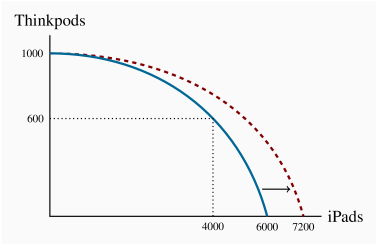EXERCISE 1.3

By examining the opportunity cost in the region where the combinations are defined, and by assuming a linear trade-off between each set of combinations, it can be seen that the first combination in the table is feasible, but not the second combination.

EXERCISE 1.4
1. $20. 2.$30.

3. See diagram.

4. See diagram.

5. The person with the lower wage.EXERCISE 1.5
1. Louis has an advantage in cutting the grass while Carrie Anne should wash cars.

2. If they each work a twelve-hour day, between them they can cut 12 lawns and wash 24 cars.

EXERCISE 1.6

Following the method described in the text:1. Carrie Anne's lawn intercept is now 12 rather than 8.

2. Yes, specialization still matters because C.A. is more efficient at cars.

3. The new coordinates will be 40 on the vertical axis, 24 on the horizontal axis and the kink point is (12,24).

EXERCISE 1.7
1. 220 cakes requires 55 workers, the remaining 45 workers can produce 135 shirts. Hence this combination lies inside the PPF described in Exercise 1.1.

2. 98 workers.

3. 2%.

### Chapter 2 Solutions

EXERCISE 2.1

These variables are positively related.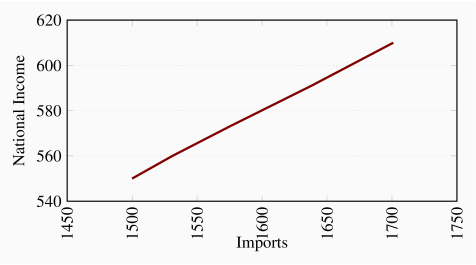EXERCISE 2.2

For (b) the answer is 30%, for (c) the answer is 4.0%, and for (d) the answers are 3.5% and -2.0%.

 Year 2005 2006 2007 2008 2009 2010 2011 2012 2013 2014 Index 100 105 109 115 120 120 127 130 139 142
EXERCISE 2.3

The scatter diagram plots observed combinations of income and consumption as follows. For parts (c) and (d): the variables are positively related and the causation runs from income to consumption.EXERCISE 2.4

The percentage changes in income are:

 Pct Inc 1.3 2.7 2 4 2.7 2 3.1 Pct Con 3 1.6 3.7 3.8 4.1 4.1 3.4
EXERCISE 2.5

The relationship given by the equation Y=10+2X when plotted has an intercept on the vertical (Y) axis of 10 and the slope of the line is 2. The maximum value of Y (where X is 12) is 34.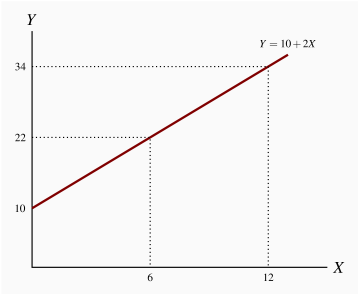X 0 1 2 3 4 5 6 7 8 9 10 11 12 Y 10 12 14 16 18 20 22 24 26 28 30 32 34
EXERCISE 2.61. The relationship is negative.

2. The relationship is non-linear.

### Chapter 3 Solutions

EXERCISE 3.1
1. The diagram shows the supply and demand curves from the data in the table. These curves intersect at the equilibrium price $32 and the equilibrium quantity 7. 2. Excess demand is 6 and excess supply is 3. 3. With excess demand the price is bid up, with excess supply the price is pushed down. 4. Equate supply P to demand: 18+2Q=60–4Q, implying 6Q=42, which is Q=7. Hence P=32.EXERCISE 3.2 1. Demand curve facing Air Canada shifts left and down. The price of the substitute Via Rail has fallen and reduced the quantity of air transport services demanded at any price. 2. Demand curve facing Air Canada shifts left and down. The substitute car travel has improved in quality and perhaps declined in cost. 3. Demand curve facing Air Canada shifts left and down. A new budget air carrier is another substitute for Air Canada that will divide the market for air transport. EXERCISE 3.3 The market diagrams are drawn on the assumption that each product can be purchased for a given price, the supply curve in each market segment is horizontal. A downward sloping demand should characterize each market. If the cigarette market is 'quashed' the demand in the market for chewing tobacco, a substitute, should shift outward, leading to higher consumption at the same price.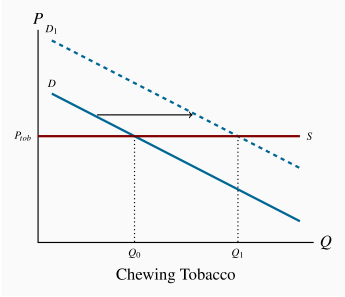EXERCISE 3.4 1. The diagram shows that equilibrium quantity is 240, equilibrium price is$130, which are the values obtained from equating supply and demand.

2. At a price of $120 the quantity demanded is 300 and the quantity supplied 210. Excess demand is therefore 90.EXERCISE 3.5 1. At a price of$140 quantity demanded is 180 and quantity supplied is 270; excess supply is therefore 90.

2. Total quotas of 180 will maintain a price of $140. This is obtained by substituting the price of$140 into the demand curve and solving for Q.

EXERCISE 3.6

It must buy 90 units at a cost of $140 each. Hence it incurs a loss on each unit of$60, making for a total loss of $5,400. EXERCISE 3.7 1. The quantity axis intercepts are 84 and 126. 2. The quantities demanded are 160, 110 and 60 respectively, on the market demand curve in the diagram. These values are obtained by solving the quantity demanded in each demand equation for a given price and summing the quantities. 3. The equation for the market demand curve is: Q=210–5P.EXERCISE 3.8EXERCISE 3.9 1. The demand curve is plotted below. 2. The supply function is horizontal as plotted. 3. Equilibrium quantity traded at P=2 is Q=225.EXERCISE 3.10 1. See the diagram below. 2. The equilibrium admission price is,. 3. The equilibrium price would now become$18 and. Yes.

4. The answer is no, because total revenue falls.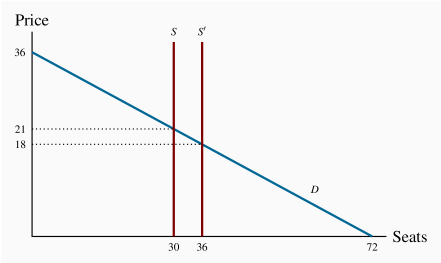EXERCISE 3.11

Wages are a cost of bringing lettuce to market. In the market diagram the supply curve for lettuce shifts upwards to reflect the increased costs. If demand is unchanged the price of lettuce rises from P0 to P1 and the quantity demanded falls from Q0 to Q1.### Chapter 4 Solutions

EXERCISE 4.1
1. Rates of growth of real GDP: